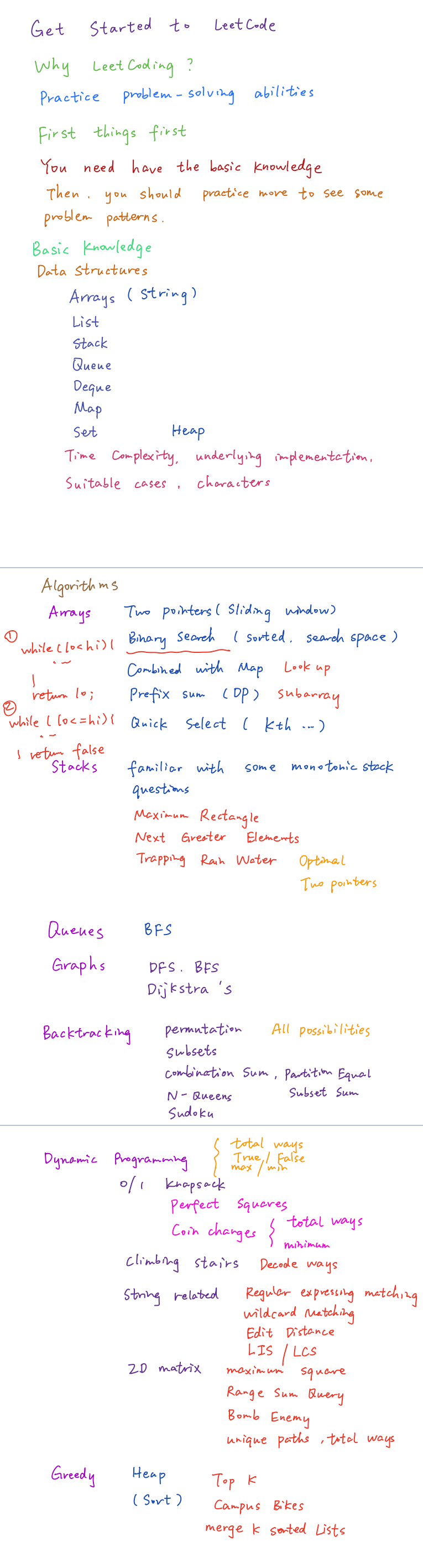# Algorithms

## Steps

1. Listen carefully, write down important information and ask for more details.
2. Draw an useful example and walk through it.
3. State a brute force.
4. Optimize:
• Unused info
• Change example
• Solve simplified problem
• Precompute
• Hash map
• Think about the best runtime
5. Walk though
6. Implement (beaufiful code)
7. Test

## Big O

• O(1): Constant, primitive operations
• O(log n): Logarithmic, reducing the size about half each time
• O(n^d) for d < 1: Sublinear
• O(n): Linear
• O(n log n): Linearithmic, divide in subproblems and merge in linear time
• O(n^2): Quadratic, nested for loops
• O(k^n): Exponential, every subset, bruteforce numerical password O(10^n)
• O(n!): Factorial, bruteforce TSP

## Approaches

### Greedy

Solve in steps making the best local decision. If the subproblem can be completed in `O(log n)`, then the Greedy strategy will be `O(n logn)` (sort and grab). If the subproblem is `O(n)` then we have `O(n^2)` (selection sort).

### Divide and Conquer

Often recursive, `O(n)` when the resolution is constant, if the resolution step is `O(n)` then we have `O(n logn)`.

### Dynamic Programming

Subdividing in simpler subproblems that are solved in a specific order and storing the results for future use. In many cases the solution is optimal.

## Problem types (source: happygirlzt)• https://leetcode.com/
• http://www.crackingthecodinginterview.com/